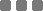## Wednesday, May 20, 2015

### JavaScript: Counting Same Occurrences in an Array

For example
We have an array:
[2, "tea", 2, 1, 2, "tea", "bag", "bag", "bag", 1]
And we wanna count the occurrence of every element (how many times it appears).

Method
There's a very long way to do that using bunch of loops.
But then, I discovered a way using `Object` construction method.
It's using just one `forEach` loop plus `if-else` conditional statement.

Steps:
1. Create an empty object.
2. Loop through the array.
3. While looping, for each of array element, create an object property of it (for that empty object).
4. The value of that object property will then be filled with array of index where the element (object property) is found.
Sounds hard, but not quite.

Let's do it

How to use it?
Let's take that array on top as the input:
[2, "tea", 2, 1, 2, "tea", "bag", "bag", "bag", 1]
So then:

The output is an `Object` which consists of
"the_array_element": [index(es)]
pairs.

If you try that snippet with the input array on your JavaScript console, you'll be seeing something like this (expand it):
To get the length for each of the property, you can do like this:

Digest
The function example above will generate an object consists of elements of the array input as the object property and the array of index(es) where each was found as the value (of the object property).

This an additional snippet to generate the exact same output as the Python equivalency post:

It consists of
"the_array_element": {"index": [index(es)], "length": index(es) length}
pairs.

To access the array of index(es) of a particular element (using the array input above), do this:
var index_tea = occurrence(array)["tea"].index;

And to access the length of a particular element:
var length_tea = occurrence(array)["tea"].length;JavaScript: Counting Same Occurrences in an Array
https://monkeyraptor.johanpaul.net/2015/05/javascript-counting-same-occurrences-in.html

#### 1 comment

1.For the getting the index specifically, it works with mine like so:
var index_tea = occurrence(array)['tea']; Where 0 is the address of occurrence in the array... for instance in your example tea occurs in indexes [ 1, 5 ] so index_tea will return 1 and not the array

Tell me what you think...